## CGAL::HalfedgeDS_decorator<HDS>

### Definition

The classes CGAL::HalfedgeDS_items_decorator<HDS>, CGAL::HalfedgeDS_decorator<HDS>, and CGAL::HalfedgeDS_const_decorator<HDS> provide additional functions to examine and to modify a halfedge data structure HDS. The class CGAL::HalfedgeDS_items_decorator<HDS> provides additional functions for vertices, halfedges, and faces of a halfedge data structure without knowing the containing halfedge data structure. The class CGAL::HalfedgeDS_decorator<HDS> stores a reference to the halfedge data structure and provides functions that modify the halfedge data structure, for example Euler-operators. The class CGAL::HalfedgeDS_const_decorator<HDS> stores a const reference to the halfedge data structure. It contains non-modifying functions, for example the test for validness of the data structure.

All these additional functions take care of the different capabilities a halfedge data structure may have or may not have. The functions evaluate the type tags of the halfedge data structure to decide on the actions. If a particular feature is not supported nothing is done. Note that for example the creation of new halfedges is mandatory for all halfedge data structures and will not appear here again.

#include <CGAL/HalfedgeDS_decorator.h>

### Creation

 HalfedgeDS_decorator D ( HDS& hds); keeps internally a reference to hds.

### Creation of New Items

 Vertex_handle D.vertices_push_back ( Vertex v) appends a copy of v to hds if vertices are supported. Returns a handle of the new vertex, or Vertex_handle() otherwise. Face_handle D.faces_push_back ( Face f) appends a copy of f to hds if faces are supported. Returns a handle of the new face, or Face_handle() otherwise.

### Creation of New Composed Items

 Halfedge_handle D.create_loop () returns handle of a halfedge from a newly created loop in hds consisting of a single closed edge, one vertex and two faces (if supported respectively). Halfedge_handle D.create_segment () returns a halfedge from a newly created segment in hds consisting of a single open edge, two vertices and one face (if supported respectively).

### Removal of Elements

The following member functions do not update affected incidence relations except if mentioned otherwise.

void D.vertices_pop_front () removes the first vertex if vertices are supported.
 Requirement: Supports_removal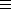CGAL::Tag_true.
void D.vertices_pop_back () removes the last vertex if vertices are supported.
void D.vertices_erase ( Vertex_handle v)
removes the vertex v if vertices are supported.
 Requirement: Supports_removalCGAL::Tag_true.
void D.vertices_erase ( Vertex_handle first, Vertex_handle last)
removes the range [first,last) if vertices are supported.
 Requirement: Supports_removalCGAL::Tag_true.

void D.faces_pop_front () removes the first face if faces are supported.
 Requirement: Supports_removalCGAL::Tag_true.
void D.faces_pop_back () removes the last face if faces are supported.
void D.faces_erase ( Face_handle f) removes the face f if faces are supported.
 Requirement: Supports_removalCGAL::Tag_true.
void D.faces_erase ( Face_handle first, Face_handle last)
removes the range [first,last) if faces are supported.
 Requirement: Supports_removalCGAL::Tag_true.

void D.erase_face ( Halfedge_handle h) removes the face incident to h from hds and changes all halfedges incident to the face into border edges or removes them from the halfedge data structure if they were already border edges. If this creates isolated vertices they get removed as well. See make_hole(h) for a more specialized variant.
 Precondition: h->is_border() == false.
 Requirement: If faces are supported, Supports_removalCGAL::Tag_true.

void D.erase_connected_component ( Halfedge_handle h)
removes the vertices, halfedges, and faces that belong to the connected component of h.
 Precondition: For all halfedges g in the connected component g.next() != Halfedge_handle().
 Requirement: Supports_removalCGAL::Tag_true.

### Modifying Functions (For Border Halfedges)

Halfedge_handle D.make_hole ( Halfedge_handle h) removes the face incident to h from hds and creates a hole.
 Precondition: h != Halfedge_handle() and !(h->is_border()).
 Requirement: If faces are supported, Supports_removalCGAL::Tag_true.

Halfedge_handle D.fill_hole ( Halfedge_handle h) fills the hole incident to h with a new face from hds. Returns h.
 Precondition: h != Halfedge_handle() and h->is_border().

Halfedge_handle D.fill_hole ( Halfedge_handle h, Face f)
fills the hole incident to h with a copy of face f. Returns h.
 Precondition: h != Halfedge_handle() and h->is_border().

Halfedge_handle D.add_face_to_border ( Halfedge_handle h, Halfedge_handle g)
extends the surface with a new face from hds into the hole incident to h and g. It creates a new edge connecting the vertex denoted by g with the vertex denoted by h and fills this separated part of the hole with a new face, such that the new face is incident to g. Returns the new halfedge that is incident to the new face.
 Precondition: h != Halfedge_handle(), g != Halfedge_handle(), h->is_border(), g->is_border() and g can be reached along the hole starting with h.

Halfedge_handle D.add_face_to_border ( Halfedge_handle h, Halfedge_handle g, Face f)
extends the surface with a copy of face f into the hole incident to h and g. It creates a new edge connecting the tip of g with the tip of h and fills this separated part of the hole with a copy of face f, such that the new face is incident to g. Returns the new halfedge that is incident to the new face.
 Precondition: h != Halfedge_handle(), g != Halfedge_handle(), h->is_border(), g->is_border() and g can be reached along the hole starting with h.

### Modifying Functions (Euler Operators)

The following Euler operations modify consistently the combinatorial structure of the halfedge data structure. The geometry remains unchanged. Note that well known graph operations are also captured with these Euler operators, for example an edge contraction is equal to a join_vertex() operation, or an edge removal to join_face().

Given a halfedge data structure hds and a halfedge handle h four special applications of the Euler operators are worth mentioning: split_vertex(h,h) results in an antenna emanating from the tip of h; split_vertex(h,h->next()->opposite()) results in an edge split of the halfedge h->next with a new vertex in-between; split_face(h,h) results in a loop directly following h; and split_face(h,h->next()) results in a bridge parallel to the halfedge h->next with a new face in-between.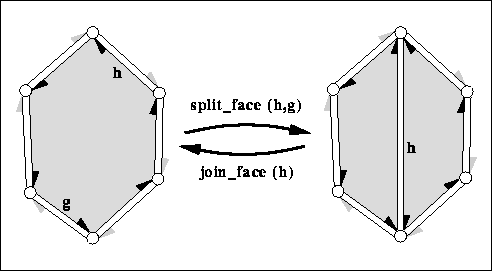Halfedge_handle D.split_face ( Halfedge_handle h, Halfedge_handle g)
splits the face incident to h and g into two faces with a new diagonal between the two vertices denoted by h and g respectively. The second (new) face obtained from hds is a copy of the first face. Returns h->next() after the operation, i.e., the new diagonal. The new face is to the right of the new diagonal, the old face is to the left. The time is proportional to the distance from h to g around the face.

Halfedge_handle D.join_face ( Halfedge_handle h) joins the two faces incident to h. The face incident to h->opposite() gets removed from hds. Both faces might be holes. Returns the predecessor of h around the face. The invariant join_face( split_face( h, g)) returns h and keeps the data structure unchanged. The time is proportional to the size of the face removed and the time to compute h->prev().
 Requirement: Supports_removalCGAL::Tag_true.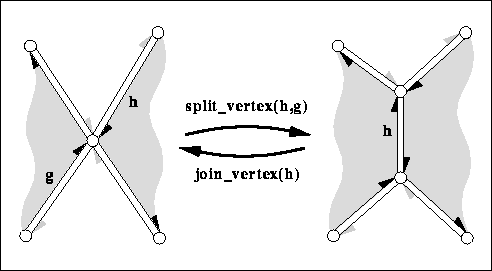Halfedge_handle D.split_vertex ( Halfedge_handle h, Halfedge_handle g)
splits the vertex incident to h and g into two vertices and connects them with a new edge. The second (new) vertex obtained from hds is a copy of the first vertex. Returns h->next()->opposite() after the operation, i.e., the new edge in the orientation towards the new vertex. The time is proportional to the distance from h to g around the vertex.

Halfedge_handle D.join_vertex ( Halfedge_handle h)
joins the two vertices incident to h. The vertex denoted by h->opposite() gets removed by hds. Returns the predecessor of h around the vertex, i.e., h->opposite()->prev(). The invariant join_vertex( split_vertex( h, g)) returns h and keeps the polyhedron unchanged. The time is proportional to the degree of the vertex removed and the time to compute h->prev() and h->opposite()->prev().
 Requirement: Supports_removalCGAL::Tag_true.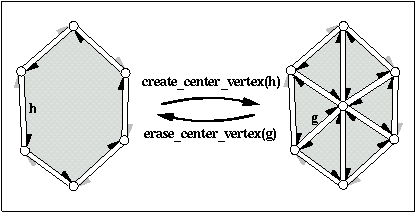Halfedge_handle D.create_center_vertex ( Halfedge_handle h)
barycentric triangulation of h->face(). Creates a new vertex, a copy of h->vertex(), and connects it to each vertex incident to h->face() splitting h->face() into triangles. h remains incident to the original face, all other triangles are copies of this face. Returns the halfedge h->next() after the operation, i.e., a halfedge pointing to the new vertex. The time is proportional to the size of the face.
 Precondition: h is not a border halfedge.

Halfedge_handle D.erase_center_vertex ( Halfedge_handle g)
reverses create_center_vertex. Erases the vertex pointed to by g and all incident halfedges thereby merging all incident faces. Only g->face() remains. The neighborhood of g->vertex() may not be triangulated, it can have larger faces. Returns the halfedge g->prev(). Thus, the invariant h == erase_center_vertex( create_center_vertex(h)) holds if h is not a border halfedge. The time is proportional to the sum of the size of all incident faces.
 Precondition: None of the incident faces of g->vertex() is a hole. There are at least two distinct faces incident to the faces that are incident to g->vertex(). (This prevents the operation from collapsing a volume into two faces glued together with opposite orientations, such as would happen with any vertex of a tetrahedron.)
 Requirement: Supports_removalCGAL::Tag_true.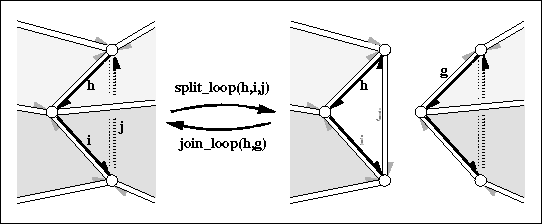Halfedge_handle D.split_loop ( Halfedge_handle h, Halfedge_handle i, Halfedge_handle j)
cuts the halfedge data structure into two parts along the cycle (h,i,j). Three new vertices (one copy for each vertex in the cycle) and three new halfedges (one copy for each halfedge in the cycle), and two new triangles are created. h,i,j will be incident to the first new triangle. The return value will be the halfedge incident to the second new triangle which is the copy of h-opposite().
 Precondition: h,i,j denote distinct, consecutive vertices of the halfedge data structure and form a cycle: i.e., h->vertex() == i->opposite()->vertex(), ..., j->vertex() == h->opposite()->vertex().

Halfedge_handle D.join_loop ( Halfedge_handle h, Halfedge_handle g)
glues the boundary of the two faces denoted by h and g together and returns h. Both faces and the vertices along the face denoted by g gets removed. Both faces may be holes. The invariant join_loop( h, split_loop( h, i, j)) returns h and keeps the data structure unchanged.
 Precondition: The faces denoted by h and g are different and have equal degree (i.e., number of edges).
 Requirement: Supports_removalCGAL::Tag_true.

### Validness Checks

These operations are the same as for CGAL::HalfedgeDS_const_decorator<HDS>.

 bool D.is_valid ( bool verbose = false, int level = 0) bool D.normalized_border_is_valid ( bool verbose = false)

### Miscellaneous

void D.inside_out () reverses face orientations.
 Precondition: is_valid() of level three.

### Example

The following program fragment illustrates the implementation of the Euler operator split_vertex() for a simplified polyhedron class.

```template <class Traits>
namespace CGAL {
class Polyhedron {
typedef HalfedgeDS_default<Traits> HDS;
HDS hds;
public:
// ...
Halfedge_handle split_vertex( Halfedge_handle h, Halfedge_handle g) {
HalfedgeDS_decorator<HDS> D(hds);
// Stricter preconditions than for HalfedgeDS only.
CGAL_precondition( D.get_vertex(h) == D.get_vertex(g));
CGAL_precondition( h != g);
return D.split_vertex( h, g);
}
};
}
```